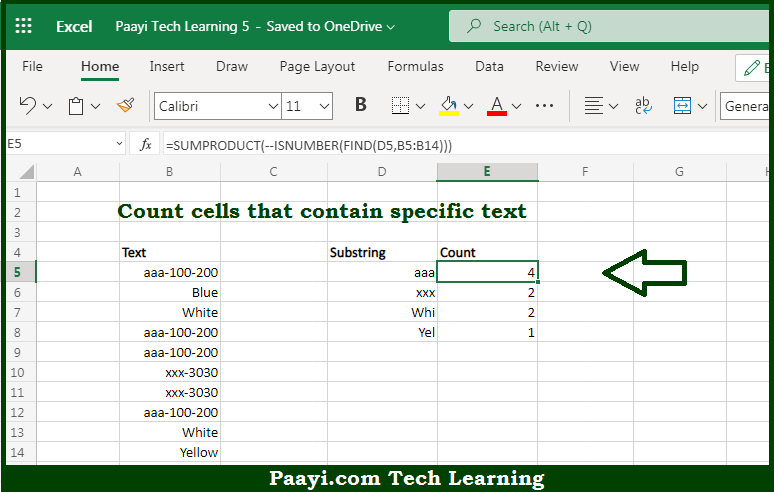# Learn How to COUNT Cells that Contain Case Sensitive Text in Microsoft Excel

Written by | 0 Comments | 580 Views

In this article, you will learn how to COUNT various things in Microsoft Excel using a single or combination of functions and its purpose in Microsoft Excel. You will also get to know how to count cells not equal to containing case sensitive text.

Count Cells that Contain Case Sensitive Text in Microsoft Excel

The main purpose of this formula is to count the cells that contain a particular text. Here we will learn how to count cells that contain case-sensitive text in Microsoft Excel.  That implies, with the help of a formula based on the  SUMPRODUCT, ISNUMBER, and FIND functions you can able count cells that contain specific text, that is text containing a substring, taking into account upper and lower case. So, with the help of this formula, you can able to count cells that contain a particular text.

General Formula to COUNT Cells that Contain Case Sensitive Text

=SUMPRODUCT(--ISNUMBER(FIND(value,data)))

The Explanation for the COUNT Cells that Contain Case Sensitive TextSo we know that with the help of the given formula above you can able to count the cells that contain a particular text. Here we will learn how to count cells that contain a case-sensitive text in Microsoft Excel. As we know that, the main purpose here is to count codes that appear as substrings in a case-sensitive way. You can use the functions COUNTIF and COUNTIFS for counting text values, but these functions are not case-sensitive, so they can't be used to solve this problem. The solution is to use the FIND function with the ISNUMBER function to check for substrings and the SUMPRODUCT function to add up the results. So, with the help of this formula, you can able to count cells that contain a case-sensitive text.